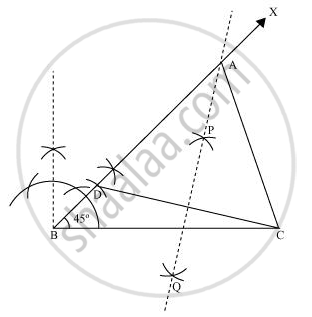# Construct a triangle ABC in which BC = 8 cm, ∠B = 45° and AB − AC = 3.5 cm. - Mathematics

Construct a triangle ABC in which BC = 8 cm, ∠B = 45° and AB − AC = 3.5 cm.

#### Solution

The below given steps will be followed to draw the required triangle.

Step I:- Draw the line segment BC = 8 cm and at point B, make an angle of 45°, say ∠XBC.

Step II:- Cut the line segment BD = 3.5 cm (equal to AB − AC) on ray BX.

Step III:- Join DC and draw the perpendicular bisector PQ of DC.

Step IV:- Let it intersect BX at point A. Join AC. ΔABC is the required triangle.Concept: Some Constructions of Triangles
Is there an error in this question or solution?

#### APPEARS IN

NCERT Class 9 Maths
Chapter 11 Constructions
Exercise 11.2 | Q 2 | Page 195